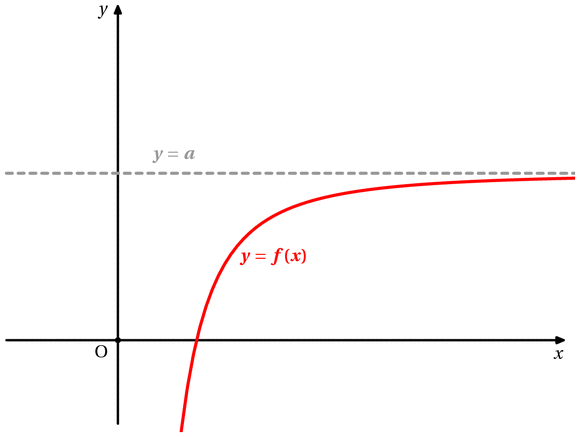Fichier f011.mp (figure 41) — Modifié le 14 Avril 2008 à 15 h 14Source

input newcourbes;
input couleur;
input geometriesyr16;
input TEX;

verbatimtex
%&latex
\documentclass{article}
\usepackage[upright]{fourier}
\usepackage{color}
\def\E{\mathrm{e}}
\let\ve\vec
\def\DR{\mathcal{D}}
\def\CR{\mathcal{C}}
\def\HR{\mathcal{H}}
\newcommand{\Mathbold}{\mbox{\boldmath$#1$\unboldmath}}
\begin{document}
etex

%%%%%%%%%%%%%%%%%%%%%%%%%%%
%
%   Asymptote horizontale
%
%%%%%%%%%%%%%%%%%%%%%%%%%%%%%%

beginfig( 41)

%%%%%%%%%%%%%%%%%%%%%%%
vardef fx(expr t)=
t
enddef;

vardef fy(expr t)=
-1/(t*t)+2                 % c'est la seule ligne à changer
enddef;
%%%%%%%%%%%%%%%%%%%%%
repere(0,0,-1,4,-1,4,2cm,1.5cm);
r_axes;
r_origine;
%r_unites;
r_labelxy;

draw f_courbe(fx,fy,.4,6,100)withpen pencircle scaled 1.5bp withcolor red;

draw r_p(-1,2)--r_p(6,2) withpen pencircle scaled 1.5bp withcolor 0.6white dashed evenly;

label.rt(btex \color{red} $\Mathbold{y=f(x)}$ etex,r_p(1,1) );
label.top(btex $\Mathbold{y=a}$ etex,r_p(.5,2) ) withcolor 0.6white;

r_fin;
endfig;

%%%%%%%%%%%%%%%%%%%%%%%%%%%
%
%   Asymptote verticale
%
%%%%%%%%%%%%%%%%%%%%%%%%%%%%%%

beginfig(42)

%%%%%%%%%%%%%%%%%%%%%%%
vardef fx(expr t)=
t
enddef;

vardef fy(expr t)=
1/((t-2))+2                 % c'est la seule ligne à changer
enddef;

vardef fz(expr t)=
1/(t-2)                % c'est la seule ligne à changer
enddef;

%%%%%%%%%%%%%%%%%%%%%
repere(0,0,-1,4,-3,8,2cm,.75cm);
r_axes;
r_origine;
%r_unites;
r_labelxy;

draw f_courbe(fx,fy,-1,1.9,100)withpen pencircle scaled 1.5bp withcolor red;
draw f_courbe(fx,fz,2.1,4,100)withpen pencircle scaled 1.5bp withcolor red;
draw r_p(2,-3)--r_p(2,8) withpen pencircle scaled 1.5bp withcolor 0.6white dashed evenly;

label.rt(btex \color{red} $\Mathbold{y=f(x)}$ etex,r_p(2.5,4) );
label.rt(btex $\Mathbold{x=a}$ etex,r_p(2,-2) ) withcolor 0.6white;

r_fin;
endfig;

%%%%%%%%%%%%%%%%%%%%%%%%%%%
%
%   Asymptote oblique
%
%%%%%%%%%%%%%%%%%%%%%%%%%%%%%%

beginfig( 43)

%%%%%%%%%%%%%%%%%%%%%%%
vardef fx(expr t)=
t
enddef;

vardef fy(expr t)=
1/(t-2)+.5*t-1        % c'est la seule ligne à changer
enddef;

%%%%%%%%%%%%%%%%%%%%%
repere(0,0,-1,6,-3,6,2cm,.75cm);
r_axes;
r_origine;
%r_unites;
r_labelxy;
label.rt(btex \color{red} $\Mathbold{y=f(x)}$ etex,r_p(2.5,4) );
label.rt(btex $\Mathbold{y=ax+b}$ etex,r_p(4,.5) ) withcolor 0.6white;
end